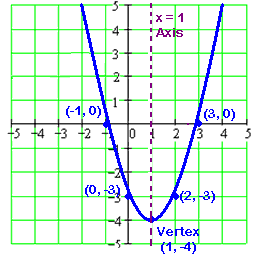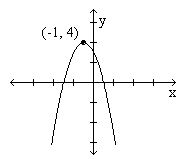# Graphing Quadratic Functions

A quadratic function may be easily sketched by finding just a few points. The most important part of the quadratic function is its vertex. Recall that the x-value of the vertex of the quadratic function, f(x) = ax² + bx + c, can be found by applying the formula, x = –b/(2a).

Quadratic functions may be sketched by finding the vertex and by finding at least two points on either side of the vertex. By finding these five points, you will always end up with a nice "∪"-shaped curve.

Example 1:

Graph the function by finding the vertex and two points on each side of the vertex.

f(x) = x² – 2x – 3

Solution:

The formula  gives the axis of symmetry and the x-value of the vertex. In this case, a = 1, b = –2, and c = –3.

 = 1

The vertex is found by substituting x = 1 into the function, f(x) = x² – 2x – 3.

f(1) = 1² – 2(1) – 3 = 1 – 2 – 3 = –4

The vertex is at the point (1, –4). This is the lowest point on the graph.

Now, go to your Table of Values and determine two points to the left of the vertex and two points to the right of the vertex.

 x y = x² – 2x – 3 y Ordered Pair –1 (–1)² – 2(–1) – 3 0 (–1, 0) 0 0² – 2(0) – 3 – 3 (0, – 3) 1 1² – 2(1) – 3 – 4 (1, –4) 2 2² – 2(2) – 3 – 3 (2, – 3) 3 3² – 2(3) – 3 0 (3, 0)Example 2:

Graph the function by finding the vertex and two points on each side of the vertex.

f(x) = 4x² – 2x

Solution:

The formula  gives the axis of symmetry and the x-value of the vertex. In this case, a = 4, b = –2, and c = 0 .

 

The vertex is found by substituting x = 1/4 into the function,f(x) = 4x² – 2x

f(1/4) = 

The vertex is at the point . This is the lowest point on the graph.

Now, go to your Table of Values and determine two points to the left of the vertex and two points to the right of the vertex.

 x y = 4x² – 2x y Ordered Pair –1 4(–1)² – 2(–1) 6 (–1, 6) 0 4(0)² – 2(0) 0 (0, 0) 1/4 4(1/4)² – 2(1/4) – 1/4  1 4(1)² – 2(1) 2 (1, 2) 2 4(2²) – 2(2) 12 (2, 12)Determine the function shown in the graph above.

A ball was kicked into the air from a balcony 20 feet above the ground, and the ball's height above the ground, in feet, t seconds after the ball was kicked was h(t) = 20 - 16t2 + 32t. What was the maximum height, in feet, of the ball above the ground after it was kicked?
Determine the vertex of the quadratic function.